# Differential equation

In mathematics, a differential equation or DE is an equation relating a function and its derivatives, the idea being that how a quantity will change is related in some way to its current value. Many of the fundamental laws of physics, chemistry, biology and economics can be formulated as differential equations. The question then becomes how to find the solutions of those equations.

The mathematical theory of differential equations has developed in parallel with the sciences where the equations originate and where the results find application. Diverse scientific fields often give rise to identical problems in differential equations. In such cases, the mathematical theory can unify otherwise quite distinct scientific fields. A celebrated example is Fourier's theory of the conduction of heat in terms of sums of trigonometric functions, Fourier series, which finds application in the propagation of sound; the propagation of electric and magnetic fields (including radio waves, visible light, X-rays, and the entire electromagnetic spectrum), as well as spectral analysis of radiation; elasticity; quantum mechanics; and many other areas of scientific research.

##  Examples

A simple differential equation is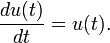$\frac{du(t)}{dt} = u(t).$

This equation is satisfied by any function which equals its derivative. One of the solutions of this equation is u(t) = et. Note that to say that a specific function (in this case et ) is a solution to a differential equation means that if you plug that function into the left-hand side of the DE and evaluate it, the result will be the right-hand side. In this case that happens since$\frac{du(t)}{dt} = \frac{d\left(e^t\right)}{dt} = e^t = u(t)\ .$

Nonlinear equations and systems of equations frequently occur in the study of physical systems. An important example of a nonlinear oscillator is the Lorenz system\begin{align} \dot{x} &= \sigma(y - x) \\ \dot{y} &= \rho x - y - x - xz \\ \dot{z} &= - \beta z + xy \end{align}

where x, y, and z are functions of t, and a dot represents the derivative with respect to t, i.e.$\dot{x}=\frac{dx(t)}{dt}\ .$

This is a basic example of a system with chaotic behavior.

The Schrödinger equation is a partial differential equation (or PDE) of fundamental importance in quantum mechanics. It governs the evolution of quantum systems and is given by$i\hbar \frac{\partial\psi(x,t)}{\partial t} = - \frac{\hbar^2}{2m} \frac{\partial^2 \psi(x,t)}{\partial x^2} + V(x,t)\psi(x,t)\ .$

Another example of a PDE is the heat equation or diffusion equation,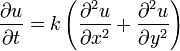$\frac{\partial u}{\partial t} = k \left(\frac{\partial^2 u}{\partial x^2} +\frac{\partial^2 u}{\partial y^2}\right)$

The reason that these two equations (the Schrödinger equation and the heat equation) are called partial differential equations is that the unknown (ψ in the Schrödinger equation, and u in the heat equation) depends on multiple variables, and the equation involves partial derivatives with respect to these variables.

The order of a differential equation is that of the highest derivative that it contains. For instance, the equation$\frac{du(t)}{dt} = u(t)$

is a first-order differential equation, while the Schrödinger equation and heat equation are examples of second order equations.

## List of differential equations

• Inhomogeneous Helmholtz equation: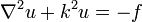$\nabla^2 u + k^2 u = -f$
• Schrödinger equation: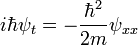$i\hbar \psi_t = - \frac{\hbar^2}{2m} \psi_{xx}$
• The simple harmonic oscillator equation: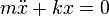$m\ddot{x}+kx=0$
• General harmonic oscillator: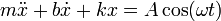$m\ddot{x}+b\dot{x}+kx=A\cos(\omega t)$
• Lotka-Volterra predator-prey: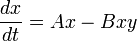$\frac{dx}{dt}=Ax-Bxy$,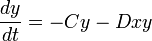$\frac{dy}{dt}=-Cy-Dxy$Some content on this page may previously have appeared on Citizendium.